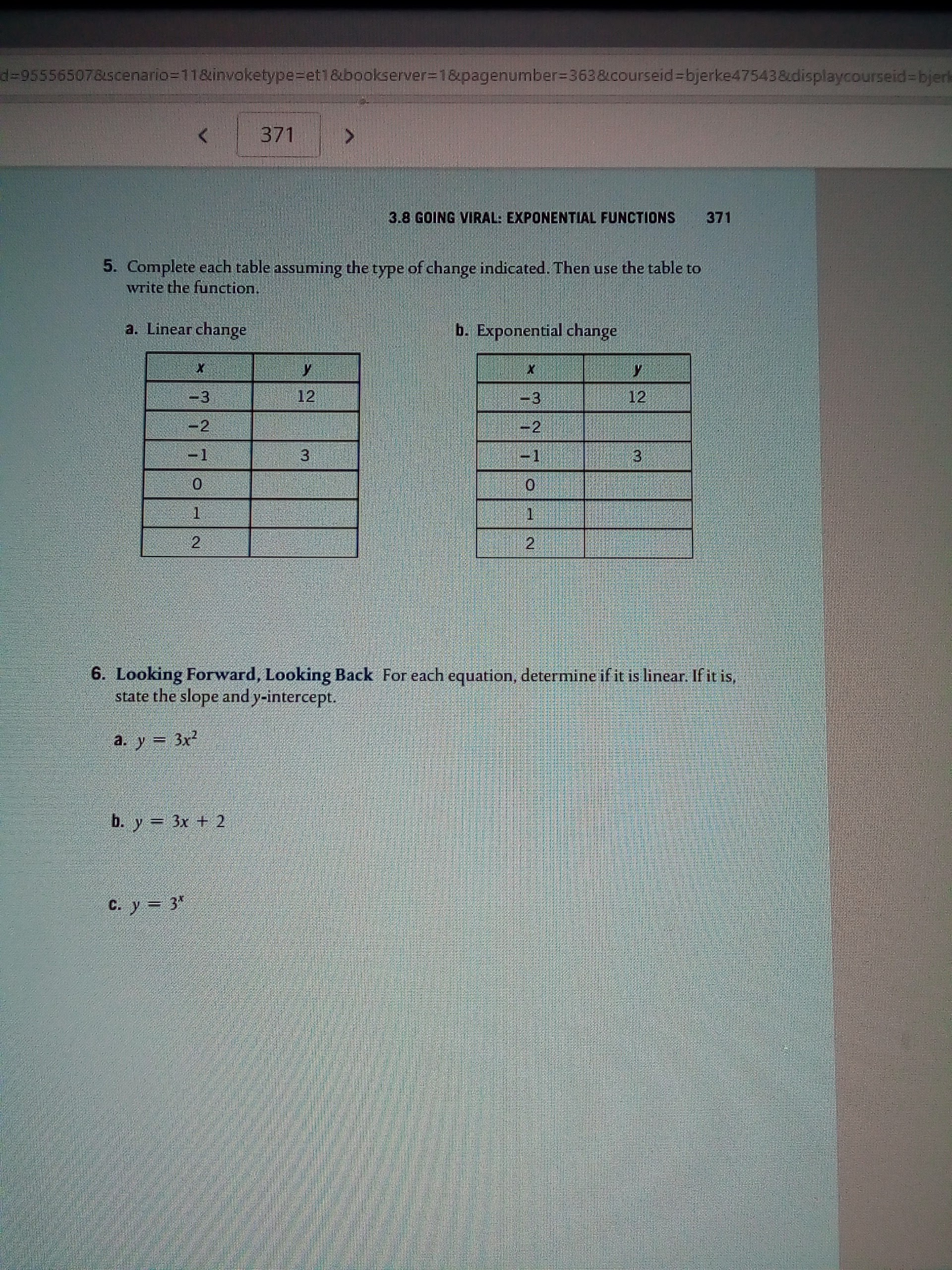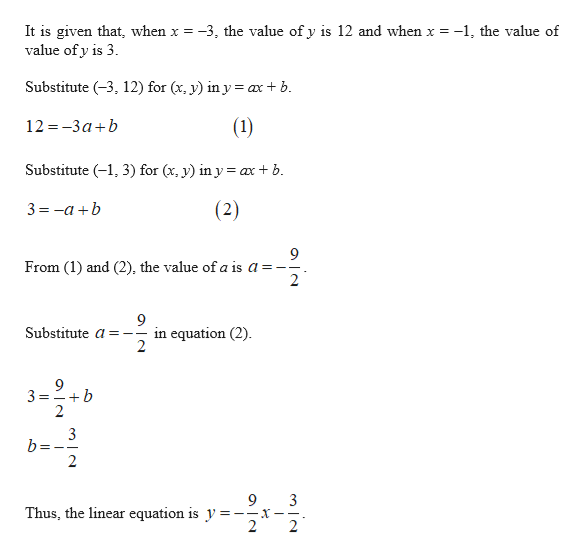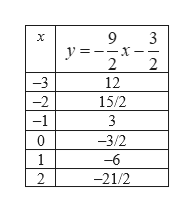# d=95556507&scenario-118invoketype-et18tbookserver=18pagenumber 363&courseid-bjerke47543 &udisplaycourseid=bjerk3713.8 GOING VIRAL: EXPONENTIAL FUNCTIONS3715. Complete each table assuming the type of change indicated. Then use the table towrite the function.a. Linear changeb. Exponential changeX123123-22-1131226. Looking Forward, Looking Back For each equation, determine if it is linear. If it is,state the slope and y-intercept.a. y 3xb. y 3x + 2C. y3

Question

How am i supposed to solve these tables without the given function? (#5)help_outlineImage Transcriptionclosed=95556507&scenario-118invoketype-et18tbookserver=18pagenumber 363&courseid-bjerke47543 &udisplaycourseid=bjerk 371 3.8 GOING VIRAL: EXPONENTIAL FUNCTIONS 371 5. Complete each table assuming the type of change indicated. Then use the table to write the function. a. Linear change b. Exponential change X 12 3 12 3 -2 2 -1 1 3 1 2 2 6. Looking Forward, Looking Back For each equation, determine if it is linear. If it is, state the slope and y-intercept. a. y 3x b. y 3x + 2 C. y3 fullscreen
check_circleExpert Solution
Step 1

(a).

Consider the linear equation is y = ax + b.help_outlineImage TranscriptioncloseIt is given that, when x = -3, the value of y is 12 and when x = -1, the value of value of y is 3 Substitute (3, 12) for (x, y) in y= ax b. (1) 12 -3ab Substitute (1, 3) for (x, y) in y = axb. 3 -ab (2) 9 From (1) and (2), the value of a is a = - 2 in equation (2) 2 Substitute a-- 3-2.-6 b 3 b= 9 3 -_X-- 2 Thus, the linear equation is y = 2 + O fullscreen
Step 2

Complete table as shown below:help_outlineImage Transcriptionclose9 3 -x 2 X y = 2 -3 12 -2 15/2 -1 3 0 -3/2 1 -6 2 -21/2 T fullscreen
Step 3

(b).

Consider the exponent...

### Want to see the full answer?

See Solution

#### Want to see this answer and more?

Solutions are written by subject experts who are available 24/7. Questions are typically answered within 1 hour*

See Solution
*Response times may vary by subject and question
Tagged in

### Other